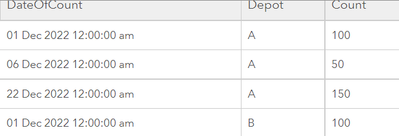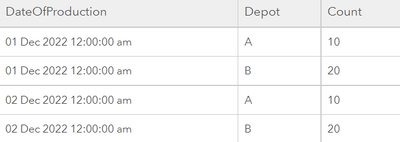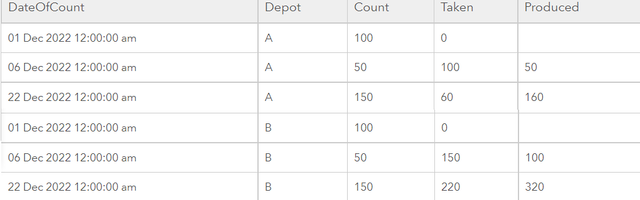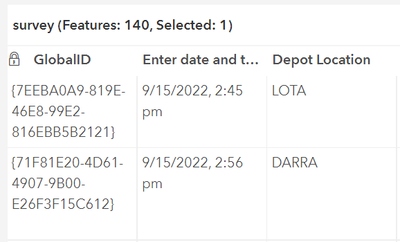# Calculating changes in a feature over different dates

123
4
12-05-2022 11:31 PMNew Contributor II

So this is potentially not possible to do with a data expression but I really do not know as this seems to be quite a complex calculation.

Consider the following table of daily sandbag counts. We are trying to dynamically count the Sandbags Taken figure based on data collected using a Survey123 count. There are 5 depots. I have been able to create a data expression that reports the latest count by depot and can use the Sum statistic on a gauge indicator to get the total current count. I am assuming that if I had the Sandbags Taken figure for each depot, I could do the same and get the grand total.

This next bit requires calculating totals for the last two dates (counts are not always daily and could be weeks apart hence needing Max(Date) and Max(Date)-1 or similar) and subtracting the total counts to calculate how many have been taken. For a bonus bit of difficulty, ideally we include the daily production figure (but currently this is in a different dataset to add a bit of complexity).

I would appreciate any thoughts on this, table below for (hopefully) some clarity.

The other alternative approach could be with Python to populate fields in the feature layer itself if a Data Expression is not going to cut it.

Len

 DateOfCount Depot Count Taken (calculated) Produced 1/11/2022 A 100 2/11/2022 A 50 50 (count Date1 – countDate2) 150 3/11/2022 A 150 50 (CountDate2+ProductionDate1-CountDate3) B B B
1 Solution

Accepted SolutionsbyMVP Regular Contributor

It is absolutely possible.

I changed your example table to reflect that the counts are not taken daily. I used the same values for depots A and B:For the production, I just assumed that depot A fills 10 sandbags per day, while depot B manages to fill 20.``````// load the count fs
var count_fs = Featureset(Text({
fields: [
{name: "DateOfCount", type: "esriFieldTypeDate"},
{name: "Depot", type: "esriFieldTypeString"},
{name: "Count", type: "esriFieldTypeInteger"},
],
features: [
{attributes: {DateOfCount: Number(Date(2022,11,1)), Depot: "A", Count: 100}},
{attributes: {DateOfCount: Number(Date(2022,11,6)), Depot: "A", Count: 50}},
{attributes: {DateOfCount: Number(Date(2022,11,22)), Depot: "A", Count: 150}},
{attributes: {DateOfCount: Number(Date(2022,11,1)), Depot: "B", Count: 100}},
{attributes: {DateOfCount: Number(Date(2022,11,6)), Depot: "B", Count: 50}},
{attributes: {DateOfCount: Number(Date(2022,11,22)), Depot: "B", Count: 150}},
],
geometryType: ""
}))
//return count_fs

var production_fs = {
fields: [
{name: "DateOfProduction", type: "esriFieldTypeDate"},
{name: "Depot", type: "esriFieldTypeString"},
{name: "Count", type: "esriFieldTypeInteger"},
],
features: [],
geometryType: ""
}
for(var i = 0; i < 30; i++) {
Push(production_fs.features, {attributes: {DateOfProduction: Number(DateAdd(Date(2022,11,1), i, "days")), Depot: "A", Count: 10}})
Push(production_fs.features, {attributes: {DateOfProduction: Number(DateAdd(Date(2022,11,1), i, "days")), Depot: "B", Count: 20}})
}
production_fs = Featureset(Text(production_fs))
//return production_fs

// create the output dict
var out = {
fields: [
{name: "DateOfCount", type: "esriFieldTypeDate"},
{name: "Depot", type: "esriFieldTypeString"},
{name: "Count", type: "esriFieldTypeInteger"},
{name: "Taken", type: "esriFieldTypeInteger"},
{name: "Produced", type: "esriFieldTypeInteger"},
],
features: [],
geometryType: ""
}

// loop over depots
var depots = ["A", "B"]
for(var d in depots) {
// find all counts of the depot and order by date
var depot = depots[d]
var depot_count_fs = Filter(count_fs, "Depot = @depot")
depot_count_fs = OrderBy(depot_count_fs, "DateOfCount")
// loop over depot_count_fs
var previous_depot_count = First(depot_count_fs)
for(var depot_count in depot_count_fs) {
// find all entries in the production_fs between now and the previous count date
var start_date = previous_depot_count.DateOfCount
var end_date = depot_count.DateOfCount
var production_between_dates = Filter(production_fs, "Depot = @depot AND DateOfProduction > @start_date AND DateOfProduction <= @end_date")
// calculate the values
var current_count = depot_count.Count
var produced = Sum(production_between_dates, "Count")
var taken = previous_depot_count.Count + produced - current_count
// append new feature
Push(out.features, {attributes: {DateOfCount: Number(depot_count.DateOfCount), Depot: depot, Count: current_count, Taken: taken, Produced: produced}})
// set previous
previous_depot_count = depot_count
}
}
return Featureset(Text(out))``````The "Taken" and "Produced" columns list the sandbags that were taken and produced between the current and the previous count.

Lines 1-35 define the sample data. They should of course be replaced with code loading your actual data sets.

Depending on your database and date format, you might have to change the sql clause in line 64.

Have a great day!
Johannes
4 RepliesbyMVP Regular Contributor

It is absolutely possible.

I changed your example table to reflect that the counts are not taken daily. I used the same values for depots A and B:For the production, I just assumed that depot A fills 10 sandbags per day, while depot B manages to fill 20.``````// load the count fs
var count_fs = Featureset(Text({
fields: [
{name: "DateOfCount", type: "esriFieldTypeDate"},
{name: "Depot", type: "esriFieldTypeString"},
{name: "Count", type: "esriFieldTypeInteger"},
],
features: [
{attributes: {DateOfCount: Number(Date(2022,11,1)), Depot: "A", Count: 100}},
{attributes: {DateOfCount: Number(Date(2022,11,6)), Depot: "A", Count: 50}},
{attributes: {DateOfCount: Number(Date(2022,11,22)), Depot: "A", Count: 150}},
{attributes: {DateOfCount: Number(Date(2022,11,1)), Depot: "B", Count: 100}},
{attributes: {DateOfCount: Number(Date(2022,11,6)), Depot: "B", Count: 50}},
{attributes: {DateOfCount: Number(Date(2022,11,22)), Depot: "B", Count: 150}},
],
geometryType: ""
}))
//return count_fs

var production_fs = {
fields: [
{name: "DateOfProduction", type: "esriFieldTypeDate"},
{name: "Depot", type: "esriFieldTypeString"},
{name: "Count", type: "esriFieldTypeInteger"},
],
features: [],
geometryType: ""
}
for(var i = 0; i < 30; i++) {
Push(production_fs.features, {attributes: {DateOfProduction: Number(DateAdd(Date(2022,11,1), i, "days")), Depot: "A", Count: 10}})
Push(production_fs.features, {attributes: {DateOfProduction: Number(DateAdd(Date(2022,11,1), i, "days")), Depot: "B", Count: 20}})
}
production_fs = Featureset(Text(production_fs))
//return production_fs

// create the output dict
var out = {
fields: [
{name: "DateOfCount", type: "esriFieldTypeDate"},
{name: "Depot", type: "esriFieldTypeString"},
{name: "Count", type: "esriFieldTypeInteger"},
{name: "Taken", type: "esriFieldTypeInteger"},
{name: "Produced", type: "esriFieldTypeInteger"},
],
features: [],
geometryType: ""
}

// loop over depots
var depots = ["A", "B"]
for(var d in depots) {
// find all counts of the depot and order by date
var depot = depots[d]
var depot_count_fs = Filter(count_fs, "Depot = @depot")
depot_count_fs = OrderBy(depot_count_fs, "DateOfCount")
// loop over depot_count_fs
var previous_depot_count = First(depot_count_fs)
for(var depot_count in depot_count_fs) {
// find all entries in the production_fs between now and the previous count date
var start_date = previous_depot_count.DateOfCount
var end_date = depot_count.DateOfCount
var production_between_dates = Filter(production_fs, "Depot = @depot AND DateOfProduction > @start_date AND DateOfProduction <= @end_date")
// calculate the values
var current_count = depot_count.Count
var produced = Sum(production_between_dates, "Count")
var taken = previous_depot_count.Count + produced - current_count
// append new feature
Push(out.features, {attributes: {DateOfCount: Number(depot_count.DateOfCount), Depot: depot, Count: current_count, Taken: taken, Produced: produced}})
// set previous
previous_depot_count = depot_count
}
}
return Featureset(Text(out))``````The "Taken" and "Produced" columns list the sandbags that were taken and produced between the current and the previous count.

Lines 1-35 define the sample data. They should of course be replaced with code loading your actual data sets.

Depending on your database and date format, you might have to change the sql clause in line 64.

Have a great day!
JohannesNew Contributor II

Hi @JohannesLindner I think you may be right that there could be a date issue in line 63 as my datecount_ field has both time and date and there could be more than one count a day (as I discovered) and I think there is an issue with some date expressions if the hours and minutes is Not Null.  I get a message Execution Error:Invalid data type for expression [date '2022-09-15 14:56:00']

Ironically this is not the first record in the table. Is there an issue if there is no previous count?Does 'Between Date1 and Date2' work from an SQL point of view?

Substituting my real fieldnames into the expression,

var portal = Portal('https://XXXXX/portal/');
var count_fs = FeatureSetByPortalItem(
portal,
'YYYYYYYYYYYYYYY',
0,
[
'datetime_count',
'field_11',
'sandbag_outside',
'sandbag_insde',
'total_note',
],
false
);
//return count_fs

var production_fs = FeatureSetByPortalItem(
portal,
'ZZZZZZZZZZZZ',
0,
[
'datetime_',
'depot_',
'produced_sandbag',
],
false
);
//return production_fs

// create the output dict
var out = {
fields: [
{name: "DateOfCount", type: "esriFieldTypeDate"},
{name: "Depot", type: "esriFieldTypeString"},
{name: "Count", type: "esriFieldTypeInteger"},
{name: "Taken", type: "esriFieldTypeInteger"},
{name: "Produced", type: "esriFieldTypeInteger"},
],
features: [],
geometryType: ""
}

// loop over depots
var depots = ["DARRA", "LOTA", "MORNINGSIDE", "NEWMARKET", "ZILLMERE"]
for(var d in depots) {
// find all counts of the depot and order by date
var depot = depots[d]
var depot_count_fs = Filter(count_fs, "field_11 = @depot")
depot_count_fs = OrderBy(depot_count_fs, "datetime_count")
// loop over depot_count_fs
var previous_depot_count = First(depot_count_fs)
for(var depot_count in depot_count_fs) {
// find all entries in the production_fs between now and the previous count date
var start_date = previous_depot_count.datetime_count
var end_date = depot_count.datetime_count
var production_between_dates = Filter(production_fs, "depot_ = @depot AND datetime_ > @start_date AND datetime_ <= @end_date")
// calculate the values
var current_count = depot_count.total_note
var produced = Sum(production_between_dates, "produced_sandbag")
var taken = previous_depot_count.total_note + produced - current_count
// append new feature
Push(out.features, {attributes: {DateOfCount: Number(depot_count.datetime_count), Depot: field_11, Count: current_count, Taken: taken, Produced: produced}})
// set previous
previous_depot_count = depot_count
}
}
return Featureset(Text(out))

Thanks again, I feel that I am nearly there after a week of trying.byMVP Regular Contributor

Does 'Between Date1 and Date2' work from an SQL point of view?

BETWEEN does work, but it includes both the start and end date, so the production values from days where you have a depot count are counted twice: once for the current count feature and once for the next. At least that's with only date values...

Ironically this is not the first record in the table.

No, but it is the first record that gets processed, because DARRA is the first depot.

There shouldn't be problems if there is no previous count, if there are multiple counts on the same day, or if there is time involved. I didn't mean to prove against those cases, but apparently I did...

I think Arcade doesn't like the SQL query. You will probably have to write it yourself, without using the @ notation.

One of these might work:

``````// Define the sql query (using one of the methods below)

// use string
var df = "Y-MM-DD HH:mm:ss"
var sql_query = `depot_ = '\${depot}' AND datetime_ > '\${Text(start_date, df)}' AND datetime_ <= '\${Text(end_date, df)}'`

// convert to sql DATE
var df = "Y-MM-DD HH:mm:ss"
var sql_query = `depot_ = '\${depot}' AND datetime_ > date '\${Text(start_date, df)}' AND datetime_ <= date '\${Text(end_date, df)}'`

// switch to full days, this might lead to small errors, like production only being applied to the next period
var df = "Y-MM-DD"
var sql_query = `depot_ = '\${depot}' AND datetime_ > '\${Text(start_date, df)}' AND datetime_ <= '\${Text(end_date, df)}'`
var sql_query = `depot_ = '\${depot}' AND datetime_ > date '\${Text(start_date, df)}' AND datetime_ <= date '\${Text(end_date, df)}'`

// use it
var production_between_dates = Filter(production_fs, sql_query)``````

If none of these work, I'd need access to the actual data to help further. Either send a public link to the service or send me the tables in a pm.

Have a great day!
JohannesNew Contributor II

Thanks so much for all your invaluable advice. I will give it a go and let you know how I get on.